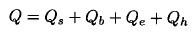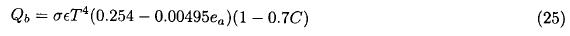APPENDIX I. HEAT FLUXES ESTIMATION

The surface heat flux computation was made based on bulk formulae. The net heat flux Q through the sea surface is given bywhere Qs, Qb, Qe, and Qh are the heat flux due to the short wave radiation, long wave radiation, latent heat, and sensible heat respectively.

The short wave radiation was estimated following Reed (1977)where C is the fraction of sky covered by clouds in tenths,is the altitude of the sun from the horizontal at noon (in degrees), A = 0.06 is the albedo,is computed with the relation sin= sin l sin[23.87 sin(2π(t–82)/365)] + cos l cos[23.87 sin(2π(t-82)/365)], where l is the latitude (Reed, 1977). The radiation under clear sky (Qc), is computed with the formulafrom Seckel and Beaudry (1973), cited in Reed (1977). In equation (21), ɸ = (2π/365)(t–21) is a function related to the day of the year in which t is the Julian day. The coefficients in equation (24) were computed following Reed (1983).

The long wave radiation was computed as Reed (1983):In this equation σ = 5.67 x 10-8 Wm–2K–4 is the Stefan Boltzman constant,is the emissivity of the sea surface, T is the temperature in degrees Kelvin, ea is the water vapor pressure (in millibars) computed following Gill (1982) and is given bywith H the percentage of relative humidity, and ew is the saturation vapor pressure computed aswhere P is the atmospheric pressure at sea level in millibars and γ = (0.7859 + 0.03477ts)/(1 + 0.00412ts), in which ts is the temperature in Celsius.

3. Latent heat flux

The latent heat flux was computed with the relationIn this relation ρa is the air density, CE is the turbulent coefficient, L is the latent heat of vaporization, w is the wind speed, qs is the saturation specific humidity and qa the specific humidity at anemometer level. The latent heat was calculated following Gill (1982) as L = 2.5008 x 106 - (2.3 x 103)Ts; ρa = 1.25 Kg m–3, qs and qa, the saturation specific humidity at sea surface and the specific humidity of air were calculated as Gill (1982):In this work, a constant value of CE = 1.4 x 10–3 is used, following Geernaert (1990).

4. Sensible heat flux

The sensible heat flux was calculated with the formulaewith CH the sensible heat turbulent coefficient, Cp is the specific heat of air, Ts the sea surface temperature, and Ta the air temperature at ship deck level. We use a constant value of CH = 1.4 x 10–3, as Geernaert (1990).